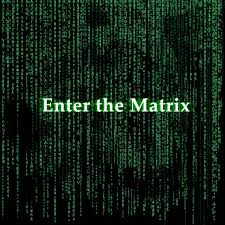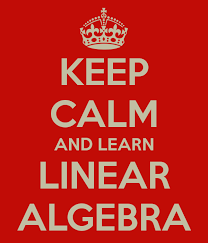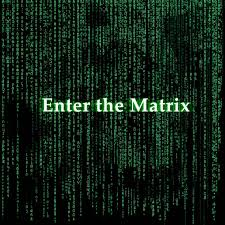Linear Algebra and Data ScienceI’m going to take a break from my last couple of posts which have been about time-series analysis in python. I’m switching gears this week to answer a question my brother asked me today at a party. I couldn’t really give him a satisfactory answer, because we were at a party. He asked me, “I’ve been studying some linear algebra. It’s a really cool subject, but it seems very abstract. I’m just having a hard time seeing how it can be applied. How does it get used in data science?” First I struggled to not do a spit take, because linear algebra is probably the most useful mathematics that I have learned to date. After that urge had passed, I fumbled around trying to verbally explain how you can use linear algebra.Okay, so for anyone that has studied any data science, and/or machine learning, knows that linear algebra is crazy useful. But I can see where somebody who does not have the background that I do might  be confused. So in this brief tutorial I’m going to give a couple of examples of how linear algebra can be useful. And we’ll write some python to make things abundantly clear.

Writing things down that humans can read

Alright, so this really isn’t an application to data science per se, but I think that it is an important point. Linear algebra let’s us talk about really complicated things. It gives us a compact way of expressing ideas that otherwise would be tedious to try to figure out what was going on. Let me give you an example. We can write a set of equations like this:$y_i=b_{0}+\sum_{j=1}^{M}b_{j}x_{i,j} \forall i \in N$

This is the formula for a linear regression, assuming that N is the set of indexes in your dataset. It looks pretty terrible. It is readable, but it hurts your eyes, and it makes it hard to really get a sense of what is going on, unless you are really familiar with this type of notation. Linear algebra can step in and simplify this notation.$\bf{Y}=\bf{Xb}$

Isn’t that just cleaner and easier to read? Y is equal to X times b. Where Y is the vector of response variables, X is the matrix of covariates and b is the vector of parameters. We now have linear algebra depiction of the same equation. But this notation just makes things simpler to read, as long as you know what is going on under the hood. But it simplifies things.

Machine Learning is Mostly Mucking About With Linear AlgebraSo OLS is probably the most simplistic machine learning model, and it amounts to finding the beta values. Okay, so for anyone that has seen how to derive parameter estimates for OLS is going to know that I am cheating a little bit in this section, because I am not going to do any calculus. If you don’t do the calculus, you can’t guarantee that this will work, but OLS is simple enough that I can get away with what I am going to do in this section. And I know that it is technically the incorrect process, so no hate mail please.

The way that this works is that we are going to simply going to take the equation for a linear regression in matrix form and simply rearrange it to solve for the beta coefficients. It turns out that if we do this, we will get the formula that we would get by doing the calculus. That happens mainly because it is linear. But in general it wouldn’t work. We just get lucky that doing so will give us the right answer. My point is that linear algebra is what machine learning is doing under the hood.

Here’s the formula again:$\bf{Y}=\bf{Xb}$

Now we want to solve for b. In normal algebra we would just divide by X and be done with it. But in linear algebra, for lots of reasons, you can only divide by a square matrix. In the case of OLS, the X matrix is almost always not square. But there is a neat trick that you can apply to transform any matrix into a square matrix. It is multiplying intself with its transpose. Depending on whether you pre or post multiply the transpose you will either get a square matrix with the size of columns or rows. Fortunately, for us this decision is made for us because the vector b is in the way and post-multiplying won’t work. One more thing to remember is that this is an equation so anything that we do to one side we have to do to the other side as well. So pre-multiplying gives us this equation:$\bf{X^{T}Y}=\bf{X^{T}Xb}$

Now$\bf{X^{T}X}$ is a square matrix so we can divide both sides by that. Which amounts to pre-multiplying both sides by its inverse. So let’s go ahead and do that:$\bf{(X^{T}X)^{-1}X^{T}Y}=\bf{(X^{T}X)^{-1}X^{T}Xb}$

And since$(X^{T}X)^{-1}X^{T}X$ is just the identity matrix we can simplify the right-hand side to just$b$ :$\bf{b}=\bf{(X^{T}X)^{-1}X^{T}Y}$

And there you go. There is the linear algebra version of the OLS equation. For those savy with statistics in linear algebra it says that b is equal to the covariance of X and Y divided by the variance of X.

So let’s write some python code so that you can see this in action. We’ll run a regression, and then we’ll write our own regression algorithm to give you the parameter values using the formula that we derived above, and then we can compare the two methods to see that statsmodels is doing this type of linear algebra under the hood.

So first we’ll import some useful libraries, namely numpy and statsmodels. Statsmodels is just for comparison purposes, for our algorithm that we develop.

import numpy as np
import statsmodels

Okay, so now we just need to generate some data. For our intercept we’ll make that a 5 and for our slope we’ll make that 15, we’ll also add in a little bit of noise to make things more realistic.

x=np.matrix([range(1000),*1000]).T
y=15*x[:,0]+5+np.random.normal()

You may be wondering what the column of 1’s is. That is our intercept column. And the numbers 0 to 999 are our X variable. So let’s see if statsmodels can recover our true coefficients of 15 and 5. Here’s the code to run OLS in statsmodels:

model=sm.OLS(endog=y,exog=x)
results=model.fit()
print(results.summary())

And here is the results of that regression:

OLS Regression Results
==============================================================================
Dep. Variable: y R-squared: 1.000
Model: OLS Adj. R-squared: 1.000
Method: Least Squares F-statistic: 1.178e+33
Date: Sun, 09 Jul 2017 Prob (F-statistic): 0.00
Time: 08:02:37 Log-Likelihood: 24829.
No. Observations: 1000 AIC: -4.965e+04
Df Residuals: 998 BIC: -4.964e+04
Df Model: 1
Covariance Type: nonrobust
==============================================================================
coef std err t P>|t| [0.025 0.975]
——————————————————————————
x1 15.0000 4.37e-16 3.43e+16 0.000 15.000 15.000
const 5.0842 2.52e-13 2.02e+13 0.000 5.084 5.084
==============================================================================
Omnibus: 793.671 Durbin-Watson: 0.001
Prob(Omnibus): 0.000 Jarque-Bera (JB): 79.767
Skew: -0.339 Prob(JB): 4.77e-18
Kurtosis: 1.793 Cond. No. 1.15e+03
==============================================================================

Warnings:
 Standard Errors assume that the covariance matrix of the errors is correctly specified.
 The condition number is large, 1.15e+03. This might indicate that there are
strong multicollinearity or other numerical problems.

Statsmodels didn’t do too bad, it has a whole bunch of extra information (which is just more linear algebra, but we won’t get into that today). Now let’s apply the formula we derived above, and see what it comes up with for parameter values. Here’s the code:

print((x.T*x).I*x.T*y)

Well, that was relatively painless. Remember .T means transpose and .I means inverse, so this is just a straight application of the formula that we derived above. Here is the result:

[[ 15.        ]
[  5.08421641]]

No big surprises here, since it is exactly the same as what statsmodels gave you. I hope that is sufficient evidence that machine learning is just (slightly more complicated than this example) linear algebra under the hood.

Markov the Grandfather of GoogleThat’s Andrey Markov. Without him, we wouldn’t have google. He came up with a really nifty idea which bears his name. The markov chain. And if you are into Bayesian statistics, we wouldn’t have the Markov Chain Monte Carlo algorithm, and bayesian statistics would be inaccessible too.

I want to finish up with just one more application of linear algebra which is really cool. It is a Markov Chain. Markov Chains are cool because they will reach an equilibrium value. It turns out that in its most simplistic form google is just calculating a bunch of equilibrium values for Markov Chains. Thank you google! If it didn’t do this we would still be stuck in a world where the internet was indexed alphabetically (which is why, when Amazon started, Jeff Bezos chose the name “Amazon”. He wanted it to show up at the top of the list!)

So let’s start off with what is a markov chain. Let’s suppose that you have a bunch of states that you can be in. To keep things concrete let’s say web pages that you are viewing. And depending on which page you are on, you are more likely to go to some pages than others next. For example, if you have a link on a page, you are more likely to click on that link and go to that page than say jump to a random page.

We can build a giant square matrix with these probabilities where the rows represent the page you are on, the columns represent the page that you are going to go to, and the elements of the matrix are the probability that the next time I look you will be at the page corresponding to the column if you were on the page corresponding to the row. That means that every row should add up to 1, which is important so that we converge to an equilibrium value. I won’t go into detail on how to derive these probabilities, just know that google obviously figured out how to do it, by collecting gobs of data when the internet was shiny and new. Let’s just assume that we staight up know the probabilities instead, and for simplicity that the internet contains only 3 pages.

Here’s some code to generate this matrix:

M=np.matrix([[0.25,0,.75],
[.2,.6,.2],
[0,.9,.1]])

So if I’m on page 1 there is a 25% chance that I will stay there, and a 75% chance I will go to page 3.

If I am on page 2 there’s a 20% chance of going to page 1 or to page 3, a 60% chance of staying on page 2.

If I am on page 3, there is a 90% chance of going to page 2 and a 10% chance of staying on page 3.

By multiplying this matrix with itself, you can “walk forward” in time. If I start off randomly on 1 of the pages and walk forward through time, where will I end up (most likely)? Here’s why it is called a chain, a square matrix multiplied by itself will be a square matrix with the same dimensions.

We can do something like this: M*M*M*M*M*M*M*M*M*M or$M^{10}$ to see where we would end up 10 steps into the future. And if you do enough of these, you will end up with a stable probability distribution of where you will be at any given time. Let’s see how our small interent will progress over time we’ll only take 3 steps into the future:

def progression(n):
for i in range(n):
print(M**(i+1))
print('')
progression(3)

And we’ll get the following output:

[[ 0.25  0.    0.75]
[ 0.2   0.6   0.2 ]
[ 0.    0.9   0.1 ]]

[[ 0.0625  0.675   0.2625]
[ 0.17    0.54    0.29  ]
[ 0.18    0.63    0.19  ]]

[[ 0.150625  0.64125   0.208125]
[ 0.1505    0.585     0.2645  ]
[ 0.171     0.549     0.28    ]]

You can see that the probabilities are already starting to converge on some values. It looks like we’ll be spending about 15% of our time on page 1, about 58% of our time on page 2 and something like 27% of our time on page 3. Indeed the final values 100s of steps in the future are pretty close to these numbers.

Google’s thought is then to display the page that everyone will end up on at the top and then the next one, and then the one after that, and so on. In our example they will give us page 2 then page 3 then page 1, in that order. Google will then let you restrict the universe of pages on the internet that you are looking at, to just pages with certain keywords. In a nutshell, if I say to google show me pages with the words “bananas that turn purple” they will do this markov chain analysis only on pages with that contain the phrase “bananas that turn purple”, and return the results in order of relevance. This is how all modern search engines work, with some tweaks here and there for efficiency.

Some Last Words on Linear Algebra

I barely scratched the surface on what linear algebra can do. But I think that this is a better answer than what I fumbled through with my brother. So hopefully, you got an appreciation for how powerful this subject in mathematics can be.

How useful is Linear Algebra, very. It is the math that I make use of the most. So yeah. Now that you have some linear algebra skills let me know in the comments how you are using linear algebra. I’d really like to know what other applications people are doing.

Oh and here is the full code for everything that we have done in python.

#!/usr/bin/env python3
# -*- coding: utf-8 -*-
"""
Created on Sun Jul  9 07:44:14 2017

@author: ryan
"""

import numpy as np
import statsmodels.api as sm

x=np.matrix([range(1000),*1000]).T
y=15*x[:,0]+5+np.random.normal()

model=sm.OLS(endog=y,exog=x)
results=model.fit()
print(results.summary())

print((x.T*x).I*x.T*y)

#%%
M=np.matrix([[0.25,0,.75],
[.2,.6,.2],
[0,.9,.1]])
def progression(n):
for i in range(n):
print(M**(i+1))
progression(10)